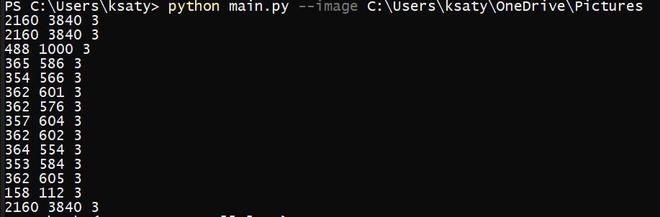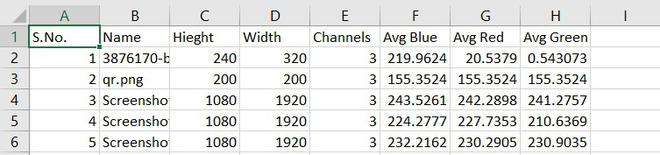# Save image properties to CSV using Python

• Difficulty Level : Expert
• Last Updated : 13 Oct, 2021

In this article, we are going to write python scripts to find the height, width, no. of channels in a given image file and save it into CSV format. Below is the implementation for the same using Python3. The prerequisite of this topic is that you have already installed NumPy and OpenCV.

Approach:

• First, we will load the required libraries into the python file ( NumPy, OpenCV, etc.).
• Create an empty CSV file with the column name only if there is no existing CSV file( Height, Width, Channels, colors, etc.). If the file data.csv does not exist then a new file will be created by the else statement.
• Now we will use argparse() function to get the directory path of the images from the user in the command line.
• Find the color properties using CV2.
• We will be using image.shape function to find out the Height, Width, Channels of the image.
• Then we will calculate the average red, average blue, average green of the image
• Then we will write the outputs to the csv file using writerow() function.

Below is the implementation:

## Python3

 `# Required Libraries ` `from` `os ``import` `listdir ` `from` `os.path ``import` `isfile, join ` `from` `pathlib ``import` `Path ` `import` `numpy ` `import` `cv2 ` `import` `argparse ` `import` `numpy ` `import` `csv ` ` `  `# Check whether the CSV  ` `# exists or not if not then create one. ` `my_file ``=` `Path(``"csv/details.csv"``) ` ` `  `if` `my_file.is_file(): ` `    ``f ``=` `open``(my_file, ``"w+"``) ` `    ``with ``open``(``'csv/details.csv'``, ``'a'``, newline``=``'') as ``file``: ` `        ``writer ``=` `csv.writer(``file``) ` `         `  `        ``writer.writerow([``"S.No."``, ``"Name"``, ``"Height"``, ` `                         ``"Width"``, ``"Channels"``, ` `                         ``"Avg Blue"``, ``"Avg Red"``, ` `                         ``"Avg Green"``]) ` `    ``f.close() ` `    ``pass` `   `  `else``: ` `    ``with ``open``(``'csv/details.csv'``, ``'w'``, newline ``=` `'') as ``file``: ` `        ``writer ``=` `csv.writer(``file``) ` `         `  `        ``writer.writerow([``"S.No."``, ``"Name"``, ``"Height"``, ` `                         ``"Width"``, ``"Channels"``, ` `                         ``"Avg Blue"``, ``"Avg Red"``, ` `                         ``"Avg Green"``]) ` ` `  `# Argparse function to get ` `# the path of the image directory ` `ap ``=` `argparse.ArgumentParser() ` ` `  `ap.add_argument(``"-i"``, ``"--image"``,  ` `                ``required ``=` `True``,  ` `                ``help` `=` `"Path to folder"``) ` ` `  `args ``=` `vars``(ap.parse_args()) ` ` `  `# Program to find the ` `# colors and embed in the CSV ` `mypath ``=` `args[``"image"``] ` ` `  `onlyfiles ``=` `[ f ``for` `f ``in` `listdir(mypath) ``if` `isfile(join(mypath,f)) ] ` `images ``=` `numpy.empty(``len``(onlyfiles), dtype ``=` `object``) ` ` `  `for` `n ``in` `range``(``0``, ``len``(onlyfiles)): ` `   `  `    ``path ``=` `join(mypath,onlyfiles[n]) ` `    ``images[n] ``=` `cv2.imread(join(mypath,onlyfiles[n]), ` `                           ``cv2.IMREAD_UNCHANGED) ` `     `  `    ``img ``=` `cv2.imread(path) ` `    ``h,w,c ``=` `img.shape ` `    ``print``(h, w, c) ` `     `  `    ``avg_color_per_row ``=` `numpy.average(img, axis ``=` `0``) ` `    ``avg_color ``=` `numpy.average(avg_color_per_row, axis ``=` `0``) ` `     `  `    ``with ``open``(``'csv/details.csv'``, ``'a'``, newline ``=` `'') as ``file``: ` `        ``writer ``=` `csv.writer(``file``) ` `        ``writer.writerow([n``+``1``, onlyfiles[n], h, w, c,  ` `                         ``avg_color[``0``], avg_color[``1``], ` `                         ``avg_color[``2``]]) ` `        ``file``.close() `

Output:### Usage:

• Save this code-named main.py.
• Shift(Key) + Right Click and Click open PowerShell window here.
`python3 main.py --image /path/to/images/folder/:`

CSV file output:Generated Csv File

My Personal Notes arrow_drop_up
Recommended Articles
Page :